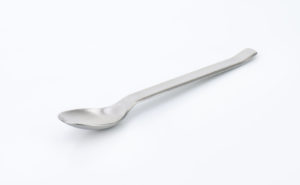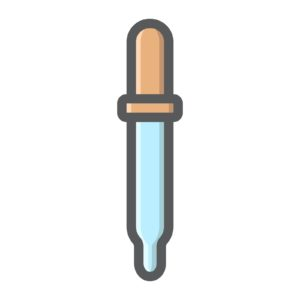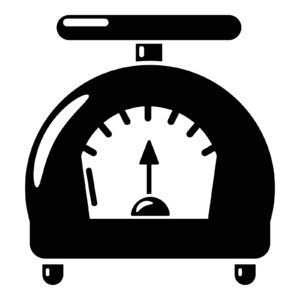## Basic Terms and Terminology Relating to Converting Within and Between Standard and Metric Systems

In this section, you will have an opportunity to master the different measurement systems including the:

• Household measurement system
• The metric measurement system
• The apothecary measurement system

## The Household Measurement SystemAlthough the household measurement system is used in homes, it is the least precise and exact of all the measurement systems. For this reason, the household measurement system is rarely used in our health care facilities but it is sometimes used for prescription that will be taken in the home after hospitalization.

The household measurement system is the system that most of us use at home, usually in the kitchen. The household system uses measurements for drops, teaspoons, tablespoons, ounces, cups, pints, quart, gallons, and pounds.There are some similarities and differences between the household system of measurements and the apothecary system of measurements. For example, a fluid ounce is the same for both the household system of measurements and the apothecary system of measurement. There are some differences as well; for example, the pound that is used to determine weight is different between these systems. In the apothecary system, there are twelve (12) ounces in a pound, and, in the household system of measurements there are sixteen (16) ounces, and not twelve (12) ounces, in the household system of measurements.

Below is a table that displays household units of measurement and their approximate equivalents in another measurement system, such as the apothecary and the metric measurement systems. It is necessary that you memorize these equivalents so that you will be able to convert from one measurement system to another.

 UNIT OF MEASUREMENT APPROXIMATE EQUIVALENT(S) 1 teaspoon 3 teaspoons = 1 tablespoon 1 tablespoon 1 tablespoon = 3 teaspoons 1 liquid ounce 1 fluid ounce = 2 tablespoons 1 ounce (weight) 16 ounces = 1 pound 1 cup 1 cup = 8 ounces 1 cup = 16 tablespoons 1 pint 1 pint = 2 cups 1 quart 1 quart = 2 pints 1 quart = 4 cups 1 gallon 1 gallon = 4 quarts 1 gallon = 8 pints 1 pound 1 pound = 16 ounces

## The Apothecary System of MeasurementThe apothecary system of measurement is one of the oldest forms of measurement. In the 1970's, the United States practically abolished this system but some still use it.

In the apothecary system, the basic measurement of weight is the grain (gr). The other forms of measurement for weight in this system include the scruple, the dram, the ounce and the pound.

For volume, the basic unit of measurement is the minim (m). A minim is equivalent to the amount of water in a drop, which is also equal to one grain. Other measurements for volume include a fluid dram, a fluid ounce, a pint, a quart and a gallon.

Lower case Roman numerals are used in this system of measurement and these Roman numerals follow the unit of measurement. For example, 4 grains is written as gr iv.

Below is a table showing the weight and volume apothecary system measures and their approximate equivalents.

 WEIGHT APPROXIMATE EQUIVALENT (S) VOLUME APPROXIMATE EQUIVALENT (S) 1 grain (gr) Weight of a grain of wheat 60 mg 1 minim Quantity of water in a drop or 1 grain 1 scruple 20 grains (gr xx) 1 fluid dram 60 minims 1 dram 3 scruples 1 fluid ounce 8 fluid drams 1 ounce 8 drams 1 pint 16 fluid ounces 1 pound 12 ounces 1 quart 2 pints 1 gallon 4 quarts

## The Metric System of Measurement

The metric system is the most commonly used measurement system around the globe with the exception of the United States.

Volume measurements are liters (L), cubic milliliters (ml) and cubic centimeter (cc). This system's volume measurements are used for oral medications, like cough syrups, and with parenteral drug dosages used intramuscularly, subcutaneously and intravenously.

The units of weight in this system include kilograms (kg), grams (g), milligrams (mg) and micrograms (mcg).

The metric system is for all the people for all the time." (Condorcet, 1791). Four everyday measuring devices that have metric calibrations: a tape measure calibrated in centimetres, a thermometer calibrated in degrees Celsius, a kilogram weight and an electrical multimeter that measures volts, amperes and ohms.

 LENGTH EQUIVALENT VOLUME EQUIVALENT WEIGHT EQUIVALENT 1 millimeter (mm) 0.001 meter 1 milliliter (mL) 0.001 liter 1 milligram (mg) 0.001 gram (g) 1 centimeter (cm) 0.01 meter 1 centiliter (cl) 0.01 liter 1 centigram (cg) 0.001 gram(g) 1 decimeter (dm) 0.1meter 1 deciliter (dl) 0.1 liter 1 decigram (dm) 0.1 gram (g) 1 kilometer (km) 1000 meters 1 kiloliter (kl) 1000 liters 1 kilogram (kg) 1000 grams (g) 1000 milliliters (mL) 1 liter 1 kilogram (kg) 2.2 pounds (lbs.) 1 milliliter (mL) cubic centimeter (cc) 1 pound (lb.) 43,592 milligrams (kg) 10 millimeters (mm) 1 centimeter (cm) 10 milliliters (mL) 1 centiliter (cl) 1 pound (lb.) 45,359.237 centigrams (cm) 10 centimeters (cm) 1 decimeter (dm) 10 centiliters (cl) 1 deciliter (dl) 1 pound (lb.) 4,535.9237 decigrams (dg) 10,000 decimeters (dm) 1 kilometer (km) 10,000 deciliters (dc) 1 kiloliter (kl)

## Converting Within and Between Measurement Systems

The table below shows conversion equivalents among the metric, apothecary and household measurement systems.

 METRIC APOTHECARY HOUSEHOLD 1 milliliter 15-16 minims 15-16 drops 4-5 milliliters 1 fluid dram 1 teaspoon or 60 drops 15-16 milliliters 4 fluid drams 1 tablespoon or 3-4 teaspoons 30 milliliters 8 fluid drams or 1 fluid ounce 2 tablespoons 240-250 milliliters 8 fluid ounces or 1/2 pint 1 glass or cup 500 milliliters 1 pint 2 glasses or 2 cups 1 liter 32 fluid ounces or 1 quart 4 glasses, 4 cups or 1 quart 1 milligram 1/60 grain 60 milligrams 1 grain 300-325 milligrams 5 grains 1 gram 15-16 grains 1 kilogram 2.2 pounds

## The Ten Most Frequently Used Conversions

The most frequently used conversions are shown below. It is suggested that you memorize these. If at any point you are not sure of a conversion factor, look it up. Do NOT under any circumstances dispense or prepare a medication that you are not certain about. Accuracy is required.

• 1 Kg = 1,000 g
• 1 Kg = 2.2 lbs.
• 1 L = 1,000 mL
• 1 g = 1,000 mg
• 1 mg = 1,000 mcg
• 1 gr = 60 mg
• 1 oz. = 30 g or 30 mL
• 1 tsp = 5 mL
• 1 lb. = 454 g
• 1 tbsp. = 15 mL

## Converting From One System of Measurement to Another

Converting one system of measurement to another system of measurement requires that you know, or you are able to look up, the equivalent unit of measurement for the different systems of measurement.

For example, if you have to convert km to miles, you will have to know how many kms in a mile or how many miles there are in a km; if you have to convert grains to grams, you will have to know how many grains in a gram or how many grams there are in a grain; and if you have to convert mg to grains, you will have to know how many mgs in a grain or how many grains there are in a mg.

Once you know the conversion equivalent, you would set up and solve an equation that can be done in a number of different ways, however, for the purpose of this review, we will use ratio and proportion as you have learned earlier in this TEAS review.

Here is an example:

If there are 2.2 pounds in one kilogram, how many kilograms are there in 160 pounds?

• Pounds : Kilograms = Pounds : Kilograms
• 2.2 Pounds : 1 Kilograms = 160 Pounds : x Kilograms
• 2.2 x = 160
• x = 160 / 2.2
• x = 72.73 kg rounded off to the nearest hundredth
• Answer - 72.73 kg is equivalent to 160 pounds

-----------

Here is another example:

If there are 2.2 pounds in one kilogram, how many pounds are there in 32 kg?

• Pounds : Kilograms = Pounds : Kilograms
• 2.2 Pounds : 1 Kilograms = x Pounds : 32 Kilograms
• x = 32 x 2.2
• x = 70.4 pounds
• Answer - 70.4 pounds is equivalent to 32 kg

----------

Here are other conversion problems examples.

Example 1:

How many mg are there in 34 grains? (1 gr = 60 mg)?

• Mg : Gr = Mg : Gr
• 60 mg : 1 gr = x mg : 34 gr
• x = 60 x 34
• x = 2040 mg
• Answer - 2040 mg is equivalent to 34 gr

----------

Example 2:

How many mL are there in 4 tablespoons? (1 tbsp. = 15 mL)

• Tbsp. : mL = Tbsp. : mL
• 1 tbsp. : 15 mL = 4 tbsp. : x mL
• x = 15 x 4
• x = 60 mL
• Answer - 60 mL is equivalent to 4 tablespoons.

----------

Example 3:

How many mL are there in 4 teaspoon? (1 tsp = 5 mL)

• Tsp : mL = Tsp : mL
• 1 tsp : 5 mL = 4 tsp : x mL
• x = 5 x 4
• x = 20 mL
• Answer - 20 mL is equivalent to 4 teaspoons.

----------

Example 4:

How many grams are there in 4 pounds? (1 lb. = 454 g)

• Pounds : Grams = Pounds : Grams
• 1 lb. : 454 g = 4 lbs. : x g
• x = 454 x 4
• x = 116 g
• Answer - 116 g is equivalent to 4 pounds.

----------

Example 5:

How many mL are there in 4.4 liters? (1000 mL = 1 Liter)

• mL : Liters = mL : Liters
• 1000 mL : 1 liter = x mL : x 4.4 liters
• x = 1000 x 4.4
• x = 4400 mL
• Answer  4400 mL is equivalent to 4.4 liters.

## Centigrade (Celsius) and Fahrenheit Temperatures

Temperatures, including environmental and bodily temperatures, can be determined and documented as either centigrade or Celsius temperatures or as Fahrenheit temperatures. The United States of America primarily uses the Fahrenheit units of temperature and the rest of the globe primarily uses the centigrade or Celsius temperature units of temperature.

Three of the primary areas where these different units of temperature are significant and important in our everyday lives are in the areas of:

• The freezing point of water
• The boiling point of water
• Bodily temperature normals

For example, the freezing point of water on the centigrade or Celsius temperature is 0 degrees C and the freezing point of water on the Fahrenheit scale is 32 degrees F.

The boiling point of water on the centigrade or Celsius temperature is 100 degrees C and the freezing point of water on the Fahrenheit scale is 32 degrees F.

Lastly, the normal body temperature for a human being on the centigrade or Celsius temperature is 37 degrees C and the normal body temperature for a human being on the Fahrenheit scale is 98.6 degrees F.

Converting temperatures from centigrade or Celsius temperature to Fahrenheit temperature is done with the following calculation.

Fahrenheit temperature = 1.8 x Celsius temperature + 32

OR

Fahrenheit temperature = 9 Celsius temperature + 32/5

Here are examples using each of the above rules or equations.

Example 1:

Convert 54 degrees Celsius to a Fahrenheit temperature.

• Fahrenheit temperature = 1.8 x Celsius temperature + 32
• Fahrenheit temperature = 1.8 x 54 +32
• Fahrenheit temperature = 97.2 + 32
• Fahrenheit temperature = 129.2 degrees F
• Answer - 129.2 degrees F is equivalent to 54 degrees Celsius

OR

Convert 54 degrees Celsius to a Fahrenheit temperature.

• Fahrenheit temperature = 9 Celsius temperature + 32/5
• Fahrenheit temperature = 9 (54) + 32/5
• Fahrenheit temperature = 97.2 + 32
• Fahrenheit temperature = 129.2 degrees F
• Answer - 129.2 degrees F is equivalent to 54 degrees Celsius

----------

Example 2:

Convert 100 degrees Celsius to a Fahrenheit temperature.

• Fahrenheit temperature = 1.8 x Celsius temperature + 32
• Fahrenheit temperature = 1.8 x 100 +32
• Fahrenheit temperature = 180 + 32
• Fahrenheit temperature = 212 degrees F
• Answer - 212 degrees F is equivalent to 54 degrees Celsius

OR

Convert 100 degrees Celsius to a Fahrenheit temperature.

• Fahrenheit temperature = 9 Celsius temperature + 32/5
• Fahrenheit temperature = 9 (100) + 32/5
• Fahrenheit temperature = 180 + 32
• Fahrenheit temperature = 212 degrees F
• Answer: 212 degrees F is equivalent to 54 degrees Celsius

Converting temperatures from Fahrenheit to centigrade or Celsius temperature is done with the following calculation.

Find the Celsius temperature when the Fahrenheit temperature is 129.2 degrees.

• Celsius temperature = Fahrenheit temperature -32 x 5/9
• Celsius temperature = 129.2 – 32 x 5/9
• Celsius temperature = 97.2 x 5/9
• Celsius temperature = 54 degrees Celsius

RELATED TEAS MEASUREMENT & DATA CONTENT:Latest posts by Alene Burke, RN, MSN (see all)